MORE IN Network Analysis
Total marks: --
Total time: --
INSTRUCTIONS
(1) Assume appropriate data and state your reasons
(2) Marks are given to the right of every question
(3) Draw neat diagrams wherever necessary

1(a) For the network shown if fig.q1(a).find the potential difference M and N using source transformation.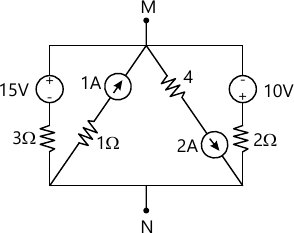4 M
1(b) Using star/delta transformation, determine the resistance between M and N of network shown in fig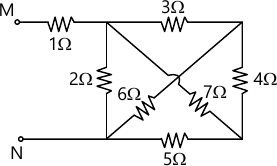4 M
1(c) For the network shown in fig.Q1(d),find power supplied by 10V source using mesh current analysis.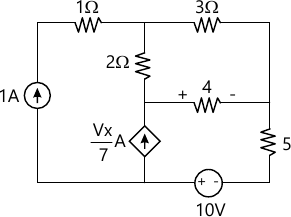6 M
1(d) For the network shown in fig.Q1(d),find the magnitude of source voltage such that current in 4 ohm is zero. Use node voltage analysis,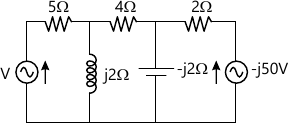6 M

2(a) Explain element - node incidence matrix with example. List the properties of the element node incidence matrix,
6 M
2(b) For the network shown in fig.. determine branch voltages.On voltage basis.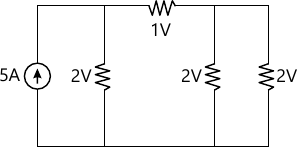8 M
2(c) Write KVL equation for the network shown. Draw the dual of this a write KCL equation and show that these two networks are dual.(fig.)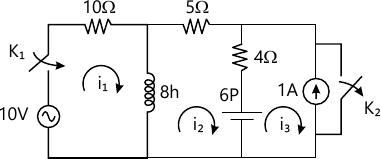6 M

3(a) Use superposition theorem to find$I_{x}$of the network shown in Fig.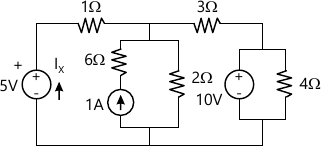8 M
3(b) For the circuit shown in Fig.Q3(b),find current 'l' using Millimon's theorem.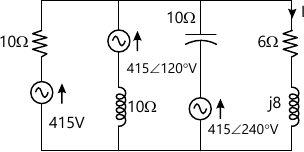6 M
3(c) State and prove reciprocity theorem
6 M

4(a) State and explain maximum power transfer when load impedance consisting of variable resistance and variable reactant.
8 M
4(b) For the network shown in Fig.Q4(b).Draw the Thevenin's equivalent circuit.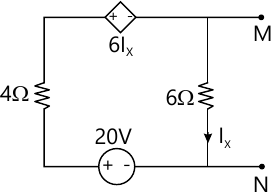5 M
4(c) Using Norton's theorem,find the current 'l' of the network shown in Fig,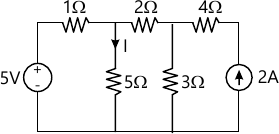7 M

5(a) What is resonance? Derive an expression for cut-off frequencies.
8 M
5(b) calculate half power frequencies of series resonant circuit where the resonance frequency is 150 Khz and band width is 75 Khz
4 M
5(c) For the circuit shown in fig.Q5(c),find two values of capacitor for the resonance.Derive the formula used take f - 50 Hz.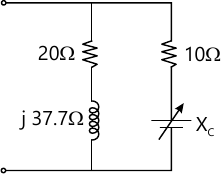8 M

6(a) What is initial and final condition? Explain the behaviour of R, l and C for the steady state condition. Determine voltage drop across swith and its first and second derivative at t= 0.
8 M
6(b) For the circuit shown in Fig. Q6(b), switch k is opened at t : 0, after reaching the steady state condition. Determine voltage drop across switch and its first and second derivative at t=0.
8 M
6(c) In the circuit shown,in fig.Q6(c),switch k is closed at t-0. Find $V_{a}(0-)\ and \ V_{a}(0+).$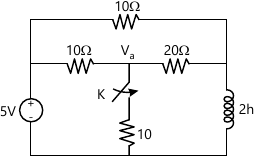6 M

7(a) For the circuit shown in Fig.Q7(a),switch 'k' is closed t-0. The initial current through inductance is 1A and initial voltage the capacitor is IV. Obtain expression for current i(-) for $t> 0.$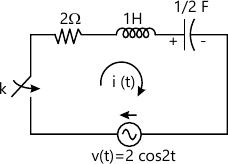8 M
7(b) For the circuit shown in Fig.Q7(b) switch is closed at t = 0. The initial current through an inductance is 2A.obtain expression for $V_{0}(+)\ for 1\geq 0.$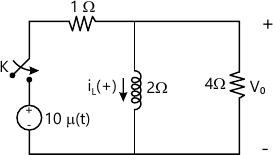6 M
7(c) synthesis the waveform shown in Fig. Q7(c) and find the Laplace transform of the periodic waveform.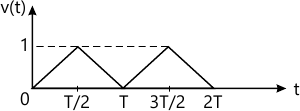6 M

8(a) Obtain transmission parameters in terms of hybrid parameters.
6 M
8(b) For the network shown in Fig Q8(b). Find the z- parameters.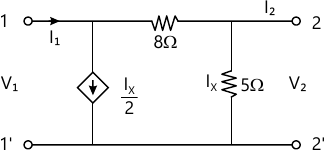8 M
8(c) Following short circuit currents and voltages are obtained experimentally for a two port network :
i) With output short circuited :[I_{1}=5 mA; I_{2}=0.3 mA and V_{1}=25V
ii) with input short circuited :[I_{1}=-5 mA; I_{2}=0.3 +10mA and V_{2}=30V].
Determine Y - parameters.
6 M

More question papers from Network Analysis